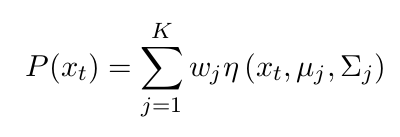# Department of Mathematics and Computer Science

www.univ-soukahras.dz/en/dept/mi

Module: Initiation à la recherché et code de déontologie

1. Information
2. Questions

## Writing Equations using Latex (1)

 1 votes What's the latex code for the following Equation:Asked on 19:26, Monday 16 May 2016 By Imed BOUCHRIKA In Initiation à la recherché et code de déontologie

 1 votes Ahlem Boulares c normalement la solution exacte :    \begin{equation} P(x_t)= \sum_{j=1}^{K}{\omega_j \eta(x_t,\mu_j,\sum_j)}\end{equation} Answered on 20:24, Tuesday 17 May 2016 by Hanen Rouainia(322 points) In Initiation à la recherché et code de déontologie